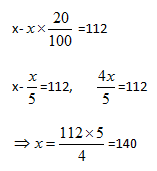# Sample Aptitude Questions of Sutherland

1. Salaries of A, B and C are in the ratio 4 : 5 : 9. If their salaries are increased by 25%, 10% and 50% respectively, what will be the new ratio of their salaries?
1. 6:5:13
2. 10:11:27
3. 5:6:15
4. 11:20:27
5. None of these

Let their original salaries be 4k, 5k and 9k.
Now, new salaries are 1.25 4k : 1.15k : 1.59k = 5k : 5.5k : 13.5k = 10:11:27
2. The ratio of the present ages of A and B 9 : 5. Five years earlier the ratio of their was 2 : 1. What is the average of their present age
1. 20 years
2. 25 years
3. 35 years
4. 40 years
5. None of these

let present age of A=9x
So present age of B=5x
According to question
9x-5/5x-5=2/1
solving this we get,
x=5 so average is 45+25/2=35 years.
1. A Shopkeeper wants to earn 12% profit on an item after giving 20% discount to the customer. By what percentage should he increase his marked price to arrive at the label price?
1. 24%
2. 32%
3. 40%
4. 16%

Let the current price be Rs. 100.
For getting 12% profit he should sell it at Rs. 112.
Let the label price be x.i.e. he need to increase 40% on label price
2. A can do a work in 60 days and B can do the same work in 40 days. They work together for 12 days and then A goes away. In how many days will B finish the remaining work?
1. 16 days
2. 20 days
3. 25 days
4. 28 days
5. 24 days

Work done by A and B in 12 days is
=12(1/60+1/40)=12 * 5/120=0.5
Remaining work = 1- [0.5]=0.5 work
B does 1/40 work in one day
so B does 0.5 work in 40 * 0.5 days = 20 days
3. A train crosses platforms of length 160 meters and 220 metres in 16 seconds and 20 seconds respectively. What is the length of the train?
1. 60 metres
2. 175 metres
3. 80 metres
4. 100 metres
5. 120 metres

Let the length of the train 'x' and speed of train be 'V' ms-1.
{160+x}/16={220+x}/20=V
{160+x}/4={220+x}/5
800+5x=880+4x;
5x-4x=880-800
x=80 metres
4. 8423 + 3120 + 6543) / (1536 + 377 + 189) =?
1. 5.8
2. 14.6
3. 8.6
4. 18.3
5. 17.2

(8423+3120+6543) is nearly equal to 18000
& (1536+377+189) is nearly equal to 2100.
So required answer is (18000) /2100 = 8.6.
5. (13% of 7439) * (3.23 % of 537) =?
1. 16243
2. 14002
3. 18674
4. 19874
5. 16774

first we calculate 13% of 7439
10% of 7439 = 744 & its 3% is nearly 224 therefore 13% of 7439 = 744 + 224 = 968.
Similarly 3.23 % of 537 = 16.2 + 1 = 17.2. So we get answer as 17.2 x 968 = 16660 (approx).
Hence option E is the answer.
6. (96)2 / 79507 1/3 = ?
1. 215
2. 259
3. 298
4. 324
5. 432

9216/43=215(app.)
7. (962)+(78961)(3/2)=?
1. 215
2. 259
3. 298
4. 324
5. 432